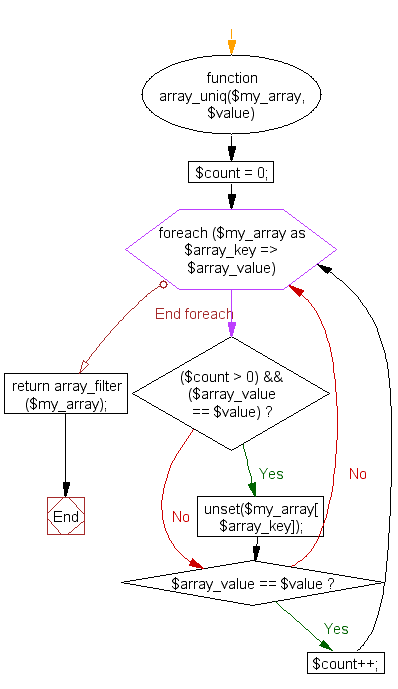﻿ PHP Array Exercise: Remove a specified duplicate entry from an array - w3resource# PHP Array Exercises : Remove a specified duplicate entry from an array

## PHP Array: Exercise-44 with Solution

Write a PHP a function to remove a specified duplicate entry from an array.

Sample Solution:

PHP Code:

``````<?php
function array_uniq(\$my_array, \$value)
{
\$count = 0;

foreach(\$my_array as \$array_key => \$array_value)
{
if ( (\$count > 0) && (\$array_value == \$value) )
{
unset(\$my_array[\$array_key]);
}

if (\$array_value == \$value) \$count++;
}

return array_filter(\$my_array);
}
\$numbers = array(4, 5, 6, 7, 4, 7, 8);

print_r(array_uniq(\$numbers, 7));
?>
```
```

Sample Output:

```Array
(
 => 4
 => 5
 => 6
 => 7
 => 4
 => 8
)
```

Flowchart:PHP Code Editor:

What is the difficulty level of this exercise?

﻿

## PHP: Tips of the Day

PHP: Extract numbers from a string

```\$str = 'In My Cart : 11 12 items';
preg_match_all('!\d+!', \$str, \$matches);
print_r(\$matches);
```